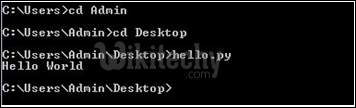# python tutorial - Variables in Python | Python Variables, Expressions, Conditions and Functions - learn python - python programming

Running your First Code in Python
Python programs are not compiled, rather they are interpreted. Now, let us move to writing a python code and running it. Please make sure that python is installed on the system you are working. If it is not installed, download it from here. We will be using python 2.7.Learn Python - Python tutorial - python sum - Python examples - Python programs

Making a Python file:
Python files are stored with the extension “.py”. Open text editor and save a file with the name “hello.py”. Open it and write the following code:

python - Sample - python code :

Linux System – Move to the directory from terminal where the created file (hello.py) is stored by using the ‘cd’ command, and then type the following in the terminal :

python - Sample - python code :

Windows system – Open command prompt and move to the directory where the file is stored by using the ‘cd’ command and then run the file by writing the file name as command.Variables in Python
Variables need not be declared first in python. They can be used directly. Variables in python are case sensitive as most of the other programming languages.
Example:

python - Sample - python code :

python tutorial - Output :

Expressions in Python
Arithmetic operations in python can be performed by using arithmetic operators and some of the in-built functions.

python - Sample - python code :

python tutorial - Output :

Conditions in Python
Conditional output in python can be obtained by using if-else and elif (else if) statements.

python - Sample - python code :

python tutorial - Output :

Functions in Python
A function in python is declared by the keyword ‘def’ before the name of the function. The return type of the function need not be specified explicitly in python. The function can be invoked by writing the function name followed by the parameter list in the brackets.

python - Sample - python code :

python tutorial - Output :

So, python is a very simplified and less cumbersome language to code in. This easiness of python is itself promoting its wide use.